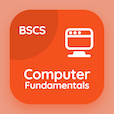Online Engineering Courses

Engineering Mathematics Practice Tests

Learn Online Engineering Mathematics Frequently Asked Questions

# Pre Employment Math Test for Engineering Jobs PDF

The e-Book Pre Employment Math Test for Engineering Jobs Multiple Choice Questions (MCQ Quiz), Engineering Mathematics Multiple Choice Questions (MCQ Quiz) PDF, download to study online engineering mathematics courses. Solve Complex Numbers and Functions Multiple Choice Questions and Answers (MCQs), Engineering Mathematics quiz answers PDF for online assessment test for jobs. The eBook Pre Employment Math Test for Engineering Jobs App Download: differential equations final exam questions with solutions, advanced maths quiz questions with answers, differential equations questions with solutions, differential equations final exam with solutions test prep for tricky trivia questions.

The MCQ: In z=r(cosθ+isinθ), r is called PDF, Pre Employment Math Test for Engineering Jobs App Download (Free) with absolute value, primary value, secondary value, and polar value choices for online assessment test for jobs. Practice pre employment math test for engineering jobs quiz questions, download Google eBook (Free Sample) for questions to ask in an interview.

## MCQs: Pre Employment Math Test for Engineering Jobs

MCQ: In z=r(cosθ+isinθ), r is called

A) absolute value
B) primary value
C) secondary value
D) polar value

MCQ: Subtraction is the inverse operation of

B) division
C) multiplication
D) inversion

MCQ: Division is the inverse operation of

B) subtraction
C) multiplication
D) inversion

MCQ: Equation z=x+iy is expressed in

A) trigonometry form
B) Laplace form
C) polar form
D) absolute form

MCQ: i2 is pure imaginary and equals to

A) 1
B) −1
C) 0.5
D) −0.5

### Practice Tests: Engineering Mathematics Exam Prep

Download Engineering Math Quiz App, Educational Psychology MCQ App, and ICT MCQs App to install for Android & iOS devices. These Apps include complete analytics of real time attempts with interactive assessments. Download Play Store & App Store Apps & Enjoy 100% functionality with subscriptions!